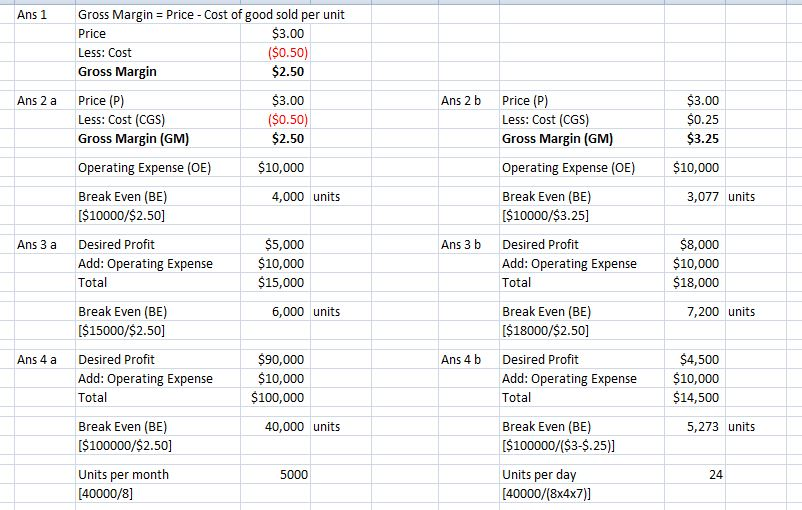Homework Help Question & Answers

# Break Even = BE             Cost of ...

Break Even = BE

Cost of Goods Sold Per Unit = CGS

Operating Expenses = OE

Price = P

Gross Margin Per Unit = GM *This number represents the amount of money per unit that can be used to contribute to cover fixed costs and add to profit.

BE = OE / (P-CGS)

GM = (P-CGS)

Use this formula to check your answers

Revenue = Expenses

Revenue = (PRICE * Quantity)

Expenses = (Fixed Costs + (CGS * Quantity)

------------------------------------------------------------------------------------------------------------

Problem 1-5 help you find the units that you would need to sell to break even assuming you knew the costs

------------------------------------------------------------------------------------------------------------

1)         So if the price of a cup of coffee at Clemens Perks costs \$3 and the CGS per unit are \$0.50 what is the Gross Margin?

GM = (P-CGS)

Answer:

2)         If the CGS per unit for coffee at Clemens Perks are \$0.50, the price is \$3, and the Operating Expenses are \$10,000/year.  How many units must Clemens Perk sell to break even in the first year?

BE = OE / (P-CGS)

Answer:

If the CGS per unit for coffee at Clemens Perk decrease to \$0.25 and everything else remains the same as above, now how many units must Clemens Perk sell to break even in the first year?

BE = OE / (P-CGS)

Answer:

3)         If Clemens Perk decided that it wants to make \$5,000 in its first year how many units must it sell to achieve this goals? (assume \$0.50 CGS)

To target a profit level

Profit Target = (OE + profit target) / (P – CGS)

This means

5k Target = (OE + 5,000) / (P – CGS)

Answer:

If Clemens Perk decides that it wants to make \$8,000 in its first year how many units must it sell to achieve this goal?  (Assume \$0.50 Variable costs)

Profit Target = (OE + profit target) / (P – CGS)

Answer:

4)         If Clemens Perk decides that it wants to make \$9,000 in its first year how many units must it sell per month (assuming they are open only 8 months) to achieve this goal?  (Assume \$0.50 Variable costs)

Profit Target = (OE + profit target) / (P – CGS)

Units / Months = Per month number

Answer:

1. If Clemens Perk decides to that it wants to make \$4,500 of profit in its first year how many units must it sell per day (assuming 8 months 4 weeks a month, 7 days a week) in order to break even?  Operating Expenses \$10,000 and CGS \$0.25/cup.

Answer:

#### Homework Answers

ReportAnswer #1

Break Even Sales: It is the volume of sales at which the total cost is equal total revenueAdd Homework Help Answer
##### Add Answer of: Break Even = BE             Cost of ...
Your Answer: Your Name: What's your source?
Not the answer you're looking for? Ask your own homework help question. Our experts will answer your question WITHIN MINUTES for Free.
More Homework Help Questions Additional questions in this topic.

• #### in a series of stamps annie must buy six of each one cost \$4.00 one cost \$2.50 one cost \$1.20 one cost \$1.00 she had to post a parcel and totals cost of postage was \$25.70

Need Online Homework Help?

Get FREE EXPERT Answers
WITHIN MINUTES
Related Questions